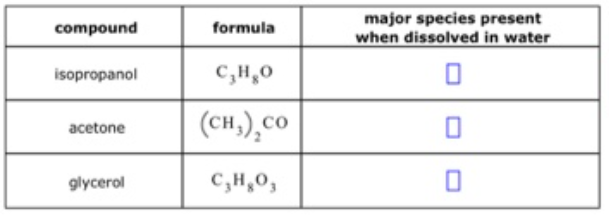# Problem: The names and chemical formulae of some chemical compounds are written in the first two columns of the table below. Each compound is soluble in water. Imagine that a few tenths of a mole of each compound is dissolved in a liter of water. Then, write down in the third column of the table the chemical formula of the major chemical species that will be present in this solution. For example, you know water itself will be present, so you can begin each list with the chemical formula for water (H2O)  Note: "major" chemical species are those present in concentrations greater than 10-6 mol/L

###### FREE Expert Solution

All the given organic molecules are soluble in water however, neither of them will dissociate like ionic compounds do.

87% (285 ratings)###### Problem Details

The names and chemical formulae of some chemical compounds are written in the first two columns of the table below. Each compound is soluble in water.

Imagine that a few tenths of a mole of each compound is dissolved in a liter of water. Then, write down in the third column of the table the chemical formula of the major chemical species that will be present in this solution. For example, you know water itself will be present, so you can begin each list with the chemical formula for water (H2O)

Note: "major" chemical species are those present in concentrations greater than 10-6 mol/LFrequently Asked Questions

What scientific concept do you need to know in order to solve this problem?

Our tutors have indicated that to solve this problem you will need to apply the Solutions, Molarity and Intermolecular Forces concept. You can view video lessons to learn Solutions, Molarity and Intermolecular Forces. Or if you need more Solutions, Molarity and Intermolecular Forces practice, you can also practice Solutions, Molarity and Intermolecular Forces practice problems.